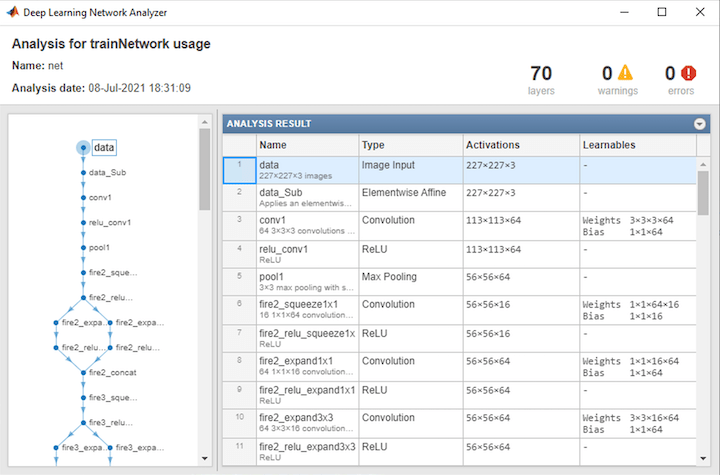Documentation

# importONNXNetwork

Import pretrained ONNX network

Import a pretrained network from ONNX™ (Open Neural Network Exchange).

By using ONNX as an intermediate format, you can import models from other deep learning frameworks that support ONNX model export, such as TensorFlow™, PyTorch, Caffe2, Microsoft® Cognitive Toolkit (CNTK), Core ML, and Apache MXNet™.## Syntax

``net = importONNXNetwork(modelfile,'OutputLayerType',outputtype)``
``net = importONNXNetwork(modelfile,'OutputLayerType',outputtype,'Classes',classes)``

## Description

````net = importONNXNetwork(modelfile,'OutputLayerType',outputtype)` imports a pretrained network from the ONNX (Open Neural Network Exchange) file `modelfile` and specifies the output layer type of the imported network.This function requires the Deep Learning Toolbox™ Converter for ONNX Model Format support package. If this support package is not installed, then the function provides a download link.```

example

````net = importONNXNetwork(modelfile,'OutputLayerType',outputtype,'Classes',classes)` additionally specifies the classes for a classification network.```

## Examples

collapse all

Download and install the Deep Learning Toolbox Converter for ONNX Model Format support package.

Type `importONNXNetwork` at the command line.

`importONNXNetwork`

If Deep Learning Toolbox Converter for ONNX Model Format is not installed, then the function provides a link to the required support package in the Add-On Explorer. To install the support package, click the link, and then click . Check that the installation is successful by importing the network from the model file `'cifarResNet.onnx'` at the command line. If the support package is installed, then the function returns a `DAGNetwork` object.

```modelfile = 'cifarResNet.onnx'; classes = ["airplane" "automobile" "bird" "cat" "dee" "dog" "frog" "horse" "ship" "truck"]; net = importONNXNetwork(modelfile,'OutputLayerType','classification','Classes',classes)```
```net = DAGNetwork with properties: Layers: [77×1 nnet.cnn.layer.Layer] Connections: [85×2 table]```

Import a residual neural network trained on the CIFAR-10 data set. Specify the file containing the ONNX network, its output type, and its output classes.

```modelfile = 'cifarResNet.onnx'; classes = ["airplane" "automobile" "bird" "cat" "deer" "dog" "frog" "horse" "ship" "truck"]; net = importONNXNetwork(modelfile, ... 'OutputLayerType','classification', ... 'Classes',classes)```
```net = DAGNetwork with properties: Layers: [77×1 nnet.cnn.layer.Layer] Connections: [85×2 table] ```

Analyze the imported network.

`analyzeNetwork(net)`## Input Arguments

collapse all

Name of ONNX model file containing the network, specified as a character vector or a string scalar. The file must be in the current folder, in a folder on the MATLAB® path, or you must include a full or relative path to the file.

Example: `'cifarResNet.onnx'`

Type of the output layer that the function appends to the end of the imported network, specified as `'classification'`, `'regression'`, or `'pixelclassification'`. Using `'pixelclassification'` appends a `pixelClassificationLayer` object (requires Computer Vision Toolbox™).

Example: `'regression'`

Classes of the output layer, specified as a categorical vector, string array, cell array of character vectors, or `'auto'`. If `Classes` is `'auto'`, then the software sets the classes to `categorical(1:N)`, where `N` is the number of classes. If you specify a string array or cell array of character vectors `str`, then the software sets the classes of the output layer to `categorical(str,str)`.

Data Types: `char` | `categorical` | `string` | `cell`

## Output Arguments

collapse all

Pretrained network, returned as `DAGNetwork` object.

## Tips

• If the ONNX network contains a layer that Deep Learning Toolbox Converter for ONNX Model Format does not support, then the function returns an error message. In this case, you can still use `importONNXLayers` to import the network architecture and weights.

`importONNXNetwork` supports the following ONNX operators, with some limitations:

• `Add`

• `AveragePool`

• `BatchNormalization`

• `Clip`

• `Concat`

• `Conv`

• `ConvTranspose`

• `Div`

• `Dropout`

• `Flatten`

• `Gemm`

• `GlobalAveragePool`

• `Identity`

• `LRN`

• `LSTM`

• `LeakyRelu`

• `MaxPool`

• `MatMul`

• `Mul`

• `PRelu`

• `Relu`

• `Reshape`

• `Sigmoid`

• `Softmax`

• `Sub`

• `Sum`

• `Tanh`

• `ImageScaler`

• To use a pretrained network for prediction or transfer learning on new images, you must preprocess your images in the same way the images that were used to train the imported model were preprocessed. Most common preprocessing steps are resizing images, subtracting image average values, and converting the images from BGR images to RGB.

• To resize images, use `imresize`. For example, `imresize(image,[227,227,3])`.

• To convert images from RGB to BGR format, use `flip`. For example, `flip(image,3)`.

For more information on preprocessing images for training and prediction, see Preprocess Images for Deep Learning.

## Compatibility Considerations

expand all

Not recommended starting in R2018b

 Open Neural Network Exchange. https://github.com/onnx/.

 ONNX. https://onnx.ai/.